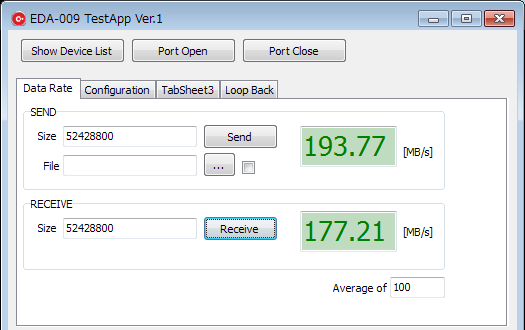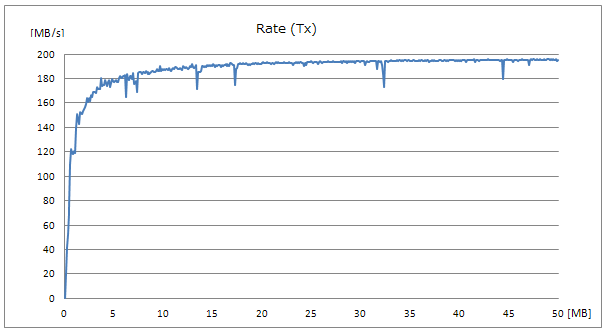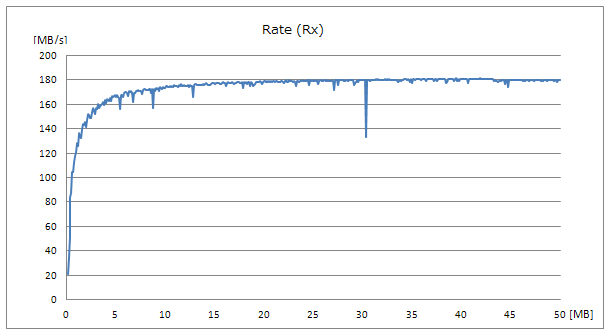# [EDA-009] Transfer rate of FTDI's USB3.0 bridge IC FT600

Last Updated: Thursday, 07 September 2017

Here is a demo of measuring transfer rate of FTDI's USB3.0 bridge IC FT600 using an EDA-009.
EDA-009 is an FPGA board which has Altera's CycloneV and FT600 on its credit-card size board.

Transfer rate of FT600 can be calculated as 200MB/s by its 16 bit data width and 100 MHz transfer frequency. An app acts actual trasfer tests and here is a result:

• Transmit : 195MB/s (average)Click to see all

Please refer to below for more details.

`Set up`

This test was done with settings below.

• FT600 bus protocol : 245 FIFO at 100MHz
• Transfered data amount  : 0.1MB (104858 Byte) to 50MB, 500 points in 0.1MB step
• Number of trials : 100
• D3XX device driver version : v1.1.0.0
• Test board : EDA-009
```Transmit Rate
```

Periods are timed between after writing data to the FT600 and asserting of complete signal by an API. The FPGA receives data wasting off of them.
Here is a part of codes of application.

```	OVERLAPPED vOverlappedWrite = {0};
vOverlappedWrite.hEvent = CreateEvent(NULL, FALSE, FALSE, NULL);

ftStatus = FT_WritePipe(ftHandle, 0x02, buff, bytesToWrite, &bytesTransferred, &vOverlappedWrite);
if (ftStatus == FT_IO_PENDING)
{
// Poll until all data requested ulBytesToWrite is sent
do
{
// FT_GetOverlappedResult will return FT_IO_INCOMPLETE if not yet finish
ftStatus  =  FT_GetOverlappedResult(ftHandle, &vOverlappedWrite,  &bytesTransferred, FALSE);
if (ftStatus == FT_IO_INCOMPLETE)
{
AttachReport(".");
num_incomp++;
continue;
}
else if(ftStatus != FT_OK)
{
CloseHandle(vOverlappedWrite.hEvent);
return -1;
}
else //if (ftStatus == FT_OK)
{
break;
}
}
while (1);
}
CloseHandle(vOverlappedWrite.hEvent);
```

Here is a state machine of the FPGA which receives data from the FT600. The clk is 200MHz. This state machine also write data to the FPGA internal FIFO memory.

```library ieee;
use ieee.std_logic_1164.all;
use ieee.std_logic_unsigned.all;

entity H_FT600_S245_RX_V3 is

port
(
clk    : in std_logic;
reset  : in std_logic;
act    : in std_logic;

rcv_cmp : out std_logic;
rcv_length : out std_logic_vector(15 downto 0);
fifo_data : out std_logic_vector(15 downto 0);
fifo_wrreq : out std_logic;

be     : in std_logic_vector(1 downto 0);
rxf    : in std_logic;
oe     : out std_logic;
rd     : out std_logic;
data   : in std_logic_vector(15 downto 0)
);

end entity;

architecture rtl of H_FT600_S245_RX_V3 is

-- Build an enumerated type for the state machine
type state_type is (idle,s0,s1,s2,s3,s4,s5,s6,t0,t1,e0,e1);
signal state : state_type;
signal rcv_length_reg : std_logic_vector(15 downto 0);
signal rxf_r : std_logic;
signal oe_r  : std_logic;
signal rd_r  : std_logic;

begin

rcv_length <= rcv_length_reg;

process (clk, reset, rxf, act)
begin
if reset = '1' then
state <= idle;
oe <= '1';
rd <= '1';
rcv_cmp <= '0';
rcv_length_reg <= X"0000";
oe_r <= '1';
rd_r <= '1';
fifo_wrreq <= '0';
elsif (rising_edge(clk)) then

rxf_r <= rxf;
oe <= oe_r;
rd <= rd_r;

case state is
when idle =>
rcv_length_reg <= X"0000";
rcv_cmp <= '0';
fifo_data <= X"BEEF";
fifo_wrreq <= '0';
oe_r <= '1';
rd_r <= '1';
if rxf_r = '0' and act = '1' then
state <= s0;
else
state <= idle;
end if;
when s0 =>
state <= s1;
when s1 =>
state <= s2;
when s2 =>
oe_r <= '0';
state <= s3;
when s3 =>
state <= s4;
when s4 =>
rd_r <= '0';
state <= s5;
when s5 =>
state <= s6;
when s6 =>
state <= t0;
---------------------------------------
when t0 =>
if rxf_r = '0' then
fifo_data <= data;
fifo_wrreq <= '1';
oe_r <= '0';
rd_r <= '0';
rcv_length_reg <= rcv_length_reg + 1;
state <= t1;
else
fifo_wrreq <= '0';
oe_r <= '1';
rd_r <= '1';
rcv_length_reg <= rcv_length_reg;
state <= e0;
end if;
when t1 =>
state <= t0;
---------------------------------------
when e0 =>
rcv_cmp <= '1';
state <= e1;
when e1 =>
rcv_cmp <= '0';
state <= idle;
end case;
end if;
end process;
end rtl;
```

An graph of transmit rate is here. It gets closer to 195MB.The FT600 suspended transmitting after sending some blocks of data.  In this test, it asserted a RXF signal after sending 0x200, 0x400, 0x600 or 0x800 amount of data words. Period of the suspends are not fixed and around from 16 to 2186 clocks.
It did not suspend transmitting within 0x200 data words. This number is same as the FT600's internal output buffer size.

`Receive Rate`

Periods are timed between requesting to receive data to the FT600 and being informed of completion.
Here is a part of codes of application.

```	overlappedRead.hEvent = CreateEventW(NULL, FALSE, FALSE, NULL);

{

if(ftStatus == FT_IO_PENDING){
while(1){
if(ftStatus == FT_IO_INCOMPLETE){
AttachReport(".");
num_incomp++;
continue;
}else if(ftStatus != FT_OK){
break;
}else{ //ftStatus == FT_OK
result = 0;
break;
}
}
}
//----------------------------------------

result = -1;
}
}else{
}
```

Here is a state machine of the FPGA which transmits  data to the FT600. The clk is 200MHz. This state machine also reads data from internal FIFO memory.

```library ieee;
use ieee.std_logic_1164.all;
use ieee.std_logic_unsigned.all;

entity H_FT600_S245_TX_V6 is

port
(
clk    : in std_logic;
reset  : in std_logic;
act    : in std_logic;

fifo_data : in std_logic_vector(15 downto 0);
fifo_rdreq : out std_logic;
fifo_empty : in std_logic;

be     : inout std_logic_vector(1 downto 0);
txe    : in std_logic;
wr     : out std_logic;
data   : inout std_logic_vector(15 downto 0);
dbg_data   : out std_logic_vector(15 downto 0)
);

end entity;

architecture rtl of H_FT600_S245_TX_V6 is

-- Build an enumerated type for the state machine
type state_type is (idle,s0,s1,s2,t0,t1,e0);
signal state : state_type;
signal fifo_rdreq_w : std_logic;
signal txe_r : std_logic;
signal wr_r : std_logic;

begin

fifo_rdreq <= fifo_rdreq_w and not txe;

process (clk, reset, txe, fifo_data)
begin
if reset = '1' then
state <= idle;
be <= (others=>'Z');
data <= (others=>'Z');
dbg_data <= X"0000";
wr <= '1';
wr_r <= '1';
fifo_rdreq_w <= '0';
elsif (rising_edge(clk)) then

txe_r <= txe;
wr <= wr_r;

case state is
when idle =>
wr_r <= '1';
data <= (others=>'Z');
be <= (others=>'Z');
fifo_rdreq_w <= '0';
if txe_r = '0' and act = '1' then
state <= s0;
else
state <= idle;
end if;
---------------------------------------------
when s0 =>
state <= s1;
when s1 =>
fifo_rdreq_w <= '1';
state <= s2;
when s2 =>
wr_r <= '0';
state <= t0;
--------------------------------------
when t0 =>
if txe_r = '0' then
fifo_rdreq_w <= '1';
wr_r <= '0';
data <= fifo_data;
be <= "11";
dbg_data <= fifo_data;
state <= t1;
else
fifo_rdreq_w <= '0';
wr_r <= '1';
data <= (others=>'Z');
be <= (others=>'Z');
dbg_data <= X"EEEE";
state <= e0;
end if;
when t1 =>
state <= t0;
----------------------------
when e0 =>
state <= idle;
end case;
------------------------------------------
end if;
end process;
end rtl;
```

An graph of receive rate is here. It gets closer to 180MB.The FT600 suspended receiving data after getting 4096 Bytes by asserting an TXE signal. This number is equal to FT600 internal buffer.

`Contact us`

We hope you find this demonstration informative.
Please let us know if you notice any mistakes.

Please be forewarned that we do not offer sample design files.

[kw] FT600 EDA-009 EDX-009 USB-106

[ ]

© HuMANDATA LTD. - Intel (Altera) & Xilinx FPGA Boards manufacturer in Japan - 2017 Sitemap